# SAT Problem of the Day SAT Problem of

• Slides: 19SAT Problem of the Day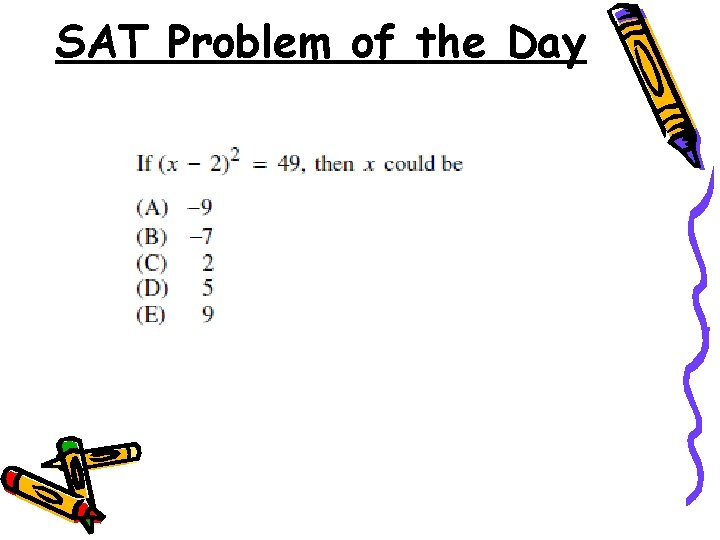SAT Problem of the Day5. 3 Factoring Quadratic Expressions Objectives: • Factor a quadratic expression • Use factoring to solve a quadratic equation and find the zeros of a quadratic functionExample 1 Factor each quadratic expression. a) 27 27 x x 2 – 18 x 9 x (3 x – 2) b) 5 x(2 x + 1) – 2(2 x + 1)( 5 x - 2 ) Factor a quadratic expression factor out the GCF for all terms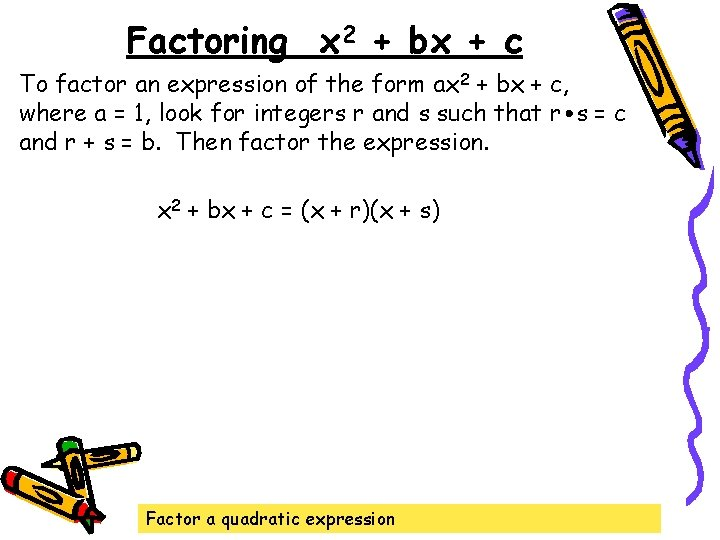Factoring x 2 + bx + c To factor an expression of the form ax 2 + bx + c, where a = 1, look for integers r and s such that r s = c and r + s = b. Then factor the expression. x 2 + bx + c = (x + r)(x + s) Factor a quadratic expressionExample 2 Factor x 2 + 12 x + 27. (x + 3)(x + 9) Factor a quadratic expressionExample 3 Factor x 2 - 15 x - 54. (x + 3)(x - 18) Factor a quadratic expression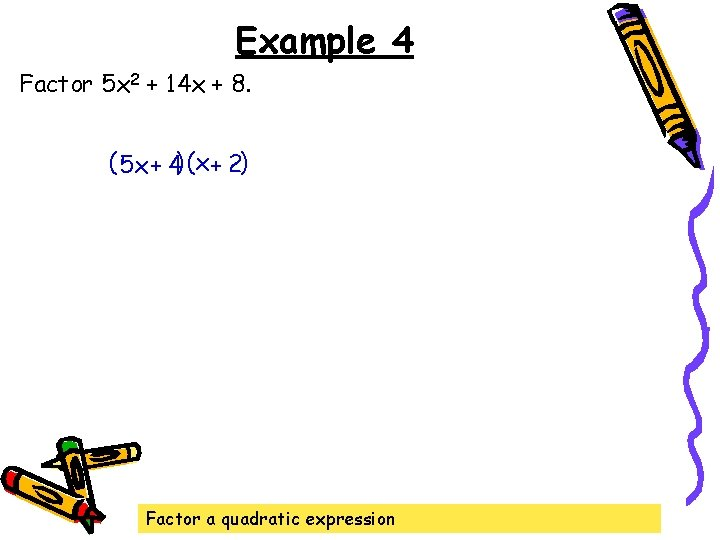Example 4 Factor 5 x 2 + 14 x + 8. ( 5 x + 4) (x + 2) Factor a quadratic expression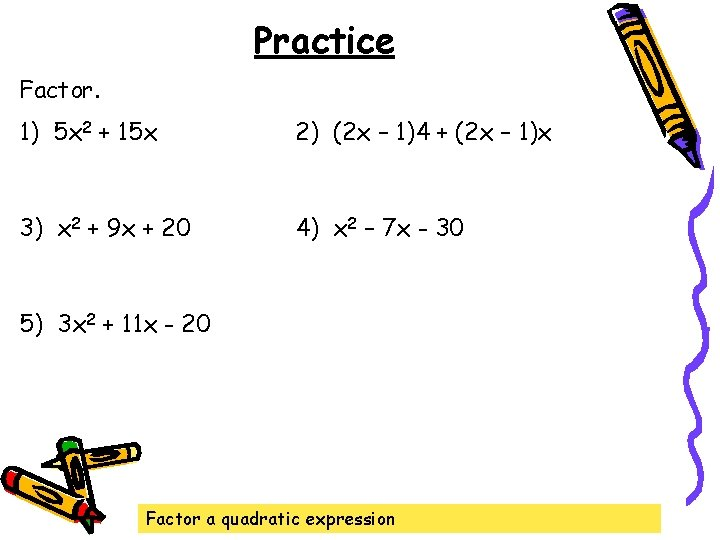Practice Factor. 1) 5 x 2 + 15 x 2) (2 x – 1)4 + (2 x – 1)x 3) x 2 + 9 x + 20 4) x 2 – 7 x - 30 5) 3 x 2 + 11 x - 20 Factor a quadratic expressionSpecial Products Factoring the Difference of Two Squares a 2 – b 2 = (a + b)(a – b) Factoring Perfect-Square Trinomials a 2 + 2 ab + b 2 = (a + b) a 2 - 2 ab + b 2 = (a - b) Factor a quadratic expression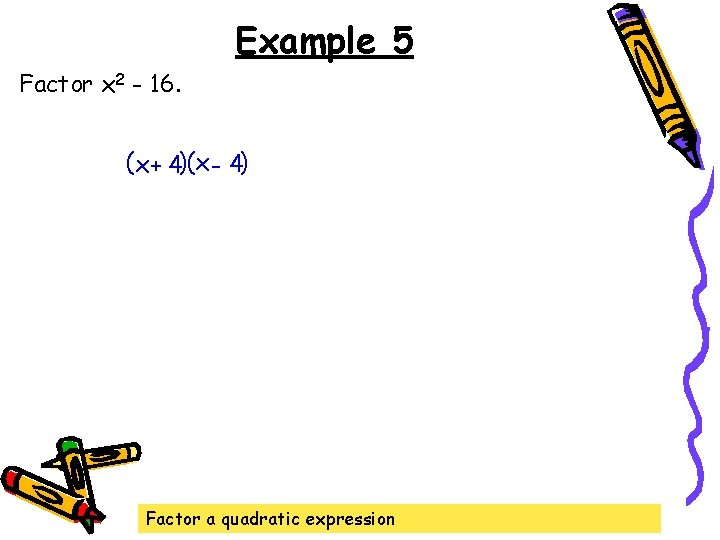Example 5 Factor x 2 - 16. (x + 4)(x - 4) Factor a quadratic expression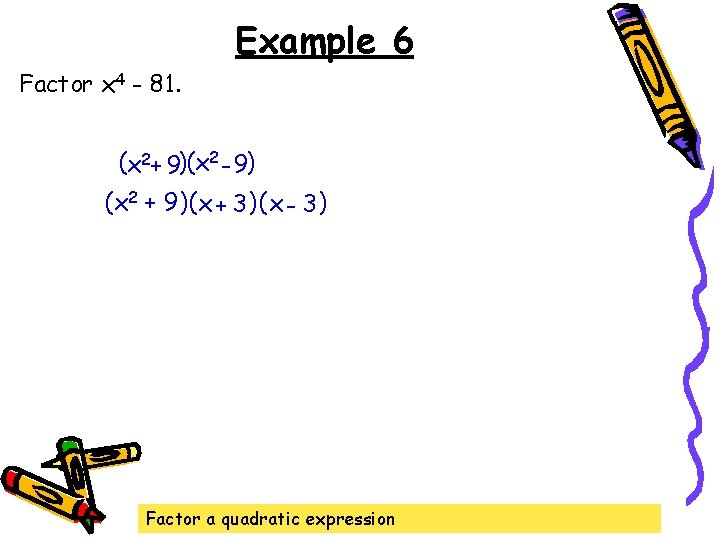Example 6 Factor x 4 - 81. (x 2+ 9)(x 2 - 9) (x 2 + 9) (x + 3 ) ( x - 3) Factor a quadratic expression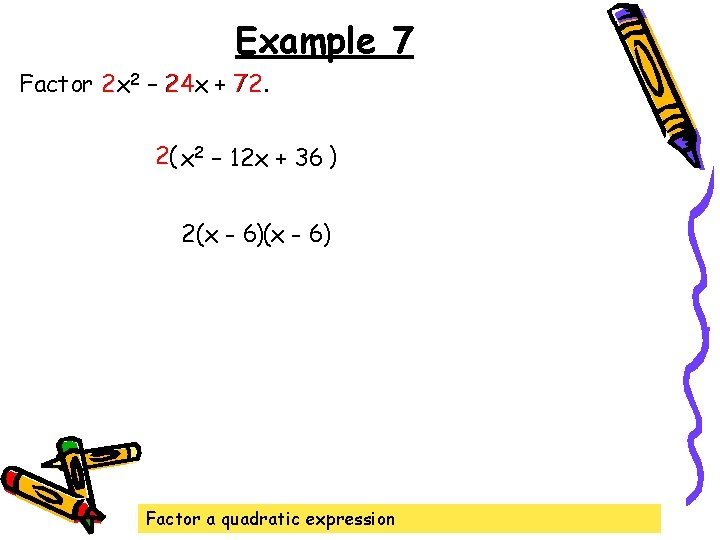Example 7 Factor 2 x 2 2 – 24 x 24 + 72 72. 2( x 2 – 12 x + 36 ) 2(x - 6) Factor a quadratic expression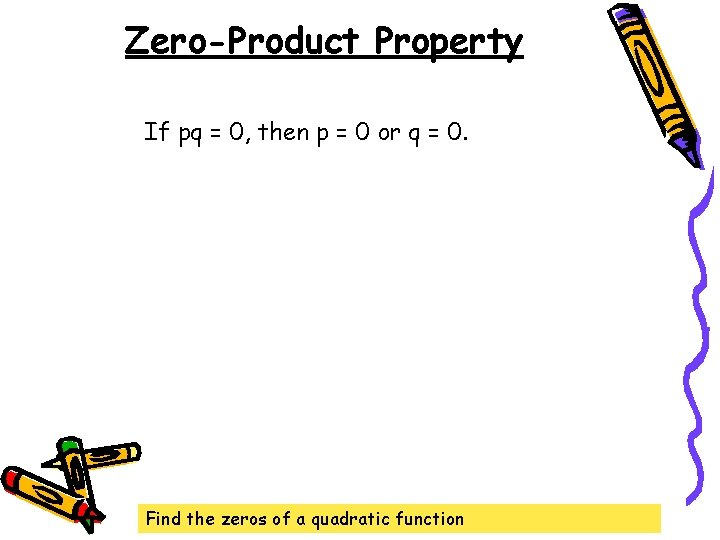Zero-Product Property If pq = 0, then p = 0 or q = 0. Find the zeros of a quadratic function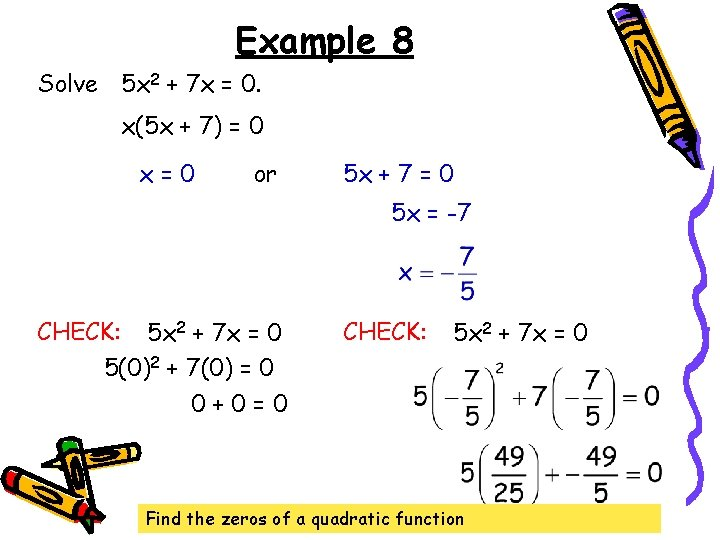Example 8 Solve 5 x 2 + 7 x = 0. x(5 x + 7) = 0 x=0 or 5 x + 7 = 0 5 x = -7 CHECK: 5 x 2 + 7 x = 0 5(0)2 + 7(0) = 0 0+0=0 CHECK: 5 x 2 + 7 x = 0 Find the zeros of a quadratic functionExample 9 Find the zeroes of the function f(x) = x 2 – 5 x + 6 = 0 (x – 3)(x – 2) = 0 x -3 = 0 x=3 CHECK: or x 2 – 4 x = x - 6 32 – 4(3) = 3 - 6 9 – 12 = -3 -3 = -3 x-2=0 x=2 CHECK: x 2 – 4 x = x - 6 22 – 4(2) = 2 - 6 4 – 8 = -4 -4 = -4 The zeroes are located at x = 2 and x = 3. Find the zeros of a quadratic function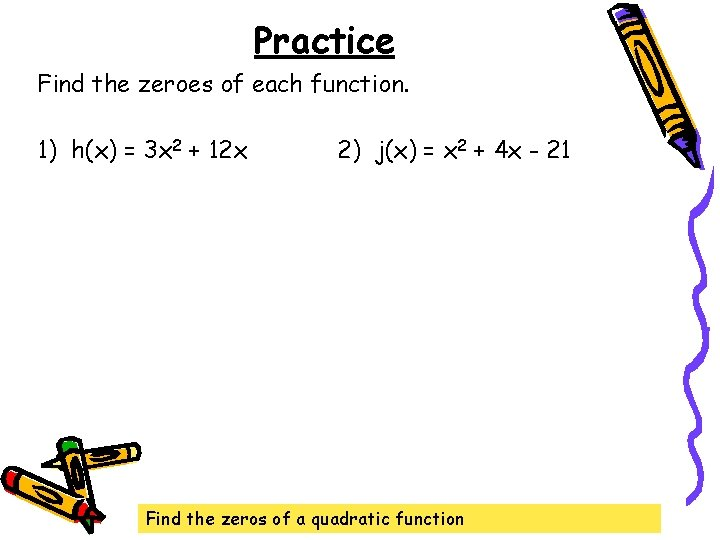Practice Find the zeroes of each function. 1) h(x) = 3 x 2 + 12 x 2) j(x) = x 2 + 4 x - 21 Find the zeros of a quadratic function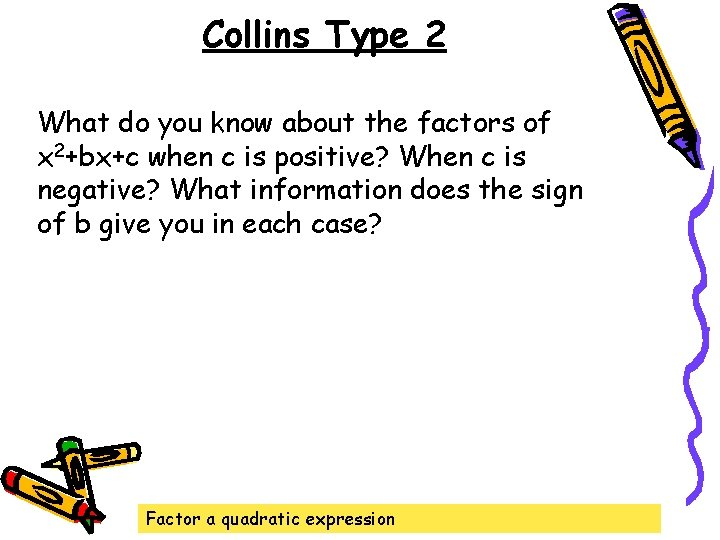Collins Type 2 What do you know about the factors of x 2+bx+c when c is positive? When c is negative? What information does the sign of b give you in each case? Factor a quadratic expressionHomework Do the problems listed on page 5 of today's packet ("Finding zeros of quadratic functions using factoring"). Some of these are exercises from Lesson 5. 3 of the textbook. Factor a quadratic expression Find the zeros of a quadratic function